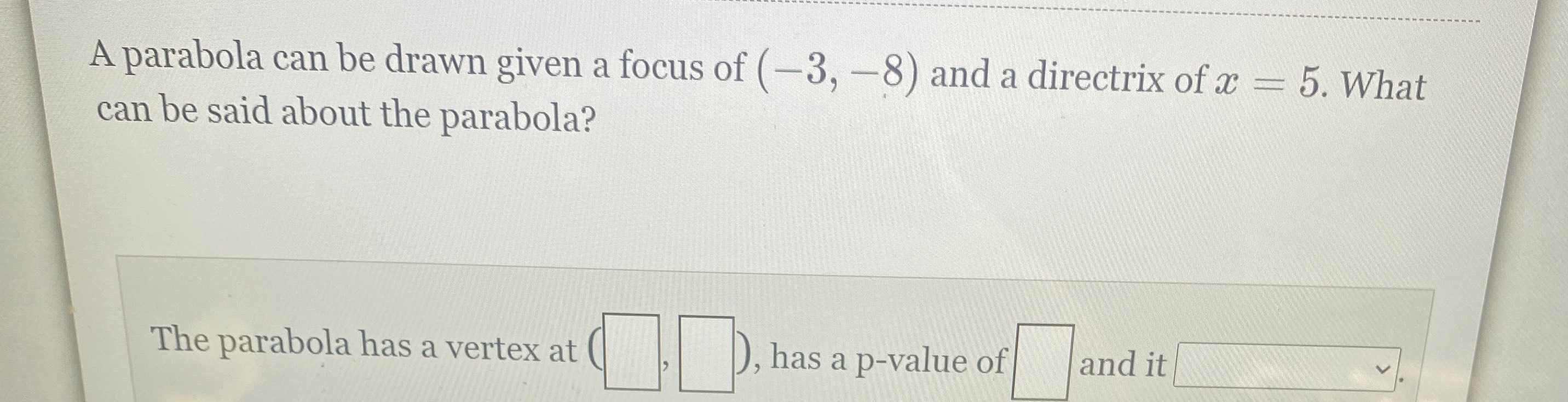### ¿Todavía tienes preguntas de matemáticas?

Pregunte a nuestros tutores expertos
Algebra
PreguntaA parabola can be drawn given a focus of $$( - 3 , - 8 )$$ and a directrix of $$x = 5 .$$ What can be said about the parabola? The parabola has a vertex at ( $$\square , \square$$ ), has a p-value of $$\square$$ and it $$\square$$ .